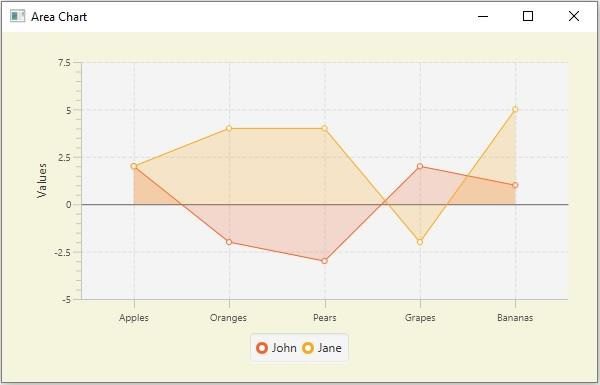# JavaFX example to create area chart with negative values

The area chart accepts a series of data points (x, y) as input values, connects them using a line, and maps the area between the obtained line and the axis. In JavaFX, you can create an area chart by instantiating the javafx.scene.chart.AreaChart class.

While instantiating this class you must pass the two objects of the Axis class representing the x and y-axis (as parameters of the constructor). Since the Axis class is abstract you need to pass objects of its concrete subclasses, NumberAxis (for numerical values) or, CategoryAxis (String values).

## Area chart with –ve values

• The XYChart.Data class represents a data point in the chart, you can create a data point by instantiating this class.

XYChart.Data dataPoint1 = new XYChart.Data(x-value, y-value)
XYChart.Data dataPoint2 = new XYChart.Data(x-value, y-value)
XYChart.Data dataPoint3 = new XYChart.Data(x-value, y-value)
• You can pass –ve integers as values (on a number axis) when you do so, a horizontal line is drawn on the (opposite) axis at 0, all the negative values are plotted below it and, all the positive values are plotted above it.

• Once you create all the required data points, you can create a series you need to instantiate the XYChart.Series class and add the data points to it.

XYChart.Series series = XYChart.Series
series.getData().add(dataPoint3);
• You can create as many numbers of such series as required.

## Example

import javafx.application.Application;
import javafx.geometry.Insets;
import javafx.scene.Scene;
import javafx.stage.Stage;
import javafx.scene.chart.AreaChart;
import javafx.scene.chart.CategoryAxis;
import javafx.scene.chart.NumberAxis;
import javafx.scene.chart.XYChart;
import javafx.scene.layout.StackPane;
public class AreaCharts_NegativeValues extends Application {
public void start(Stage stage) {
//Defining the x and y axes
CategoryAxis xAxis = new CategoryAxis();
NumberAxis yAxis = new NumberAxis(-5, 7.5, 2.5);
//Setting labels for the axes
yAxis.setLabel("Values");
//Creating an area chart
AreaChart<String, Number> areaChart = new AreaChart<String, Number>(xAxis, yAxis);
//Preparing the data points for the series1
XYChart.Series series1 = new XYChart.Series();
//Preparing the data points for the series3
XYChart.Series series2 = new XYChart.Series();
//Setting the name to all the series
series1.setName("John");
series2.setName("Jane");
//Setting the data to area chart
//Creating a stack pane to hold the chart
StackPane pane = new StackPane(areaChart);
pane.setStyle("-fx-background-color: BEIGE");
//Setting the Scene
Scene scene = new Scene(pane, 595, 350);
stage.setTitle("Area Chart");
stage.setScene(scene);
stage.show();
}
public static void main(String args[]){
launch(args);
}
}

## Output